Analytic Signals and Hilbert Transform Filters Next  |  Prev  |  Up  |  Top  |  Index  |  JOS Index  |  JOS Pubs  |  JOS Home  |  Search

### Analytic Signals and Hilbert Transform Filters

A signal which has no negative-frequency components is called an analytic signal.4.12 Therefore, in continuous time, every analytic signal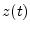can be represented as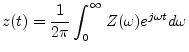where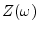is the complex coefficient (setting the amplitude and phase) of the positive-frequency complex sinusoid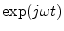at frequency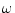.

Any real sinusoid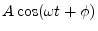may be converted to a positive-frequency complex sinusoid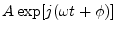by simply generating a phase-quadrature component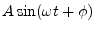to serve as the imaginary part'':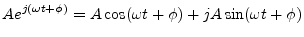The phase-quadrature component can be generated from the in-phase component by a simple quarter-cycle time shift.4.13

For more complicated signals which are expressible as a sum of many sinusoids, a filter can be constructed which shifts each sinusoidal component by a quarter cycle. This is called a Hilbert transform filter. Let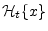denote the output at time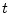of the Hilbert-transform filter applied to the signal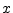. Ideally, this filter has magnitude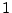at all frequencies and introduces a phase shift of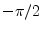at each positive frequency and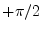at each negative frequency. When a real signal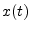and its Hilbert transform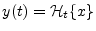are used to form a new complex signal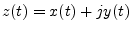, the signalis the (complex) analytic signal corresponding to the real signal. In other words, for any real signal, the corresponding analytic signal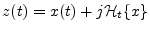has the property that all negative frequencies'' ofhave been filtered out.''

To see how this works, recall that these phase shifts can be impressed on a complex sinusoid by multiplying it by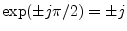. Consider the positive and negative frequency components at the particular frequency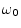: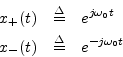Now let's apply a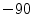degrees phase shift to the positive-frequency component, and a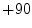degrees phase shift to the negative-frequency component: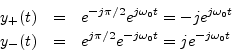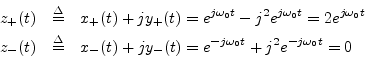and sure enough, the negative frequency component is filtered out. (There is also a gain of 2 at positive frequencies which we can remove by defining the Hilbert transform filter to have magnitude 1/2 at all frequencies.)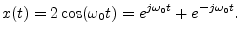Applying the ideal phase shifts, the Hilbert transform is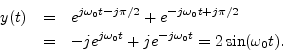The analytic signal is then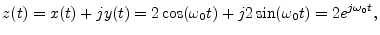by Euler's identity. Thus, in the sum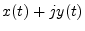, the negative-frequency components ofand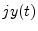cancel out, leaving only the positive-frequency component. This happens for any real signal, not just for sinusoids as in our example.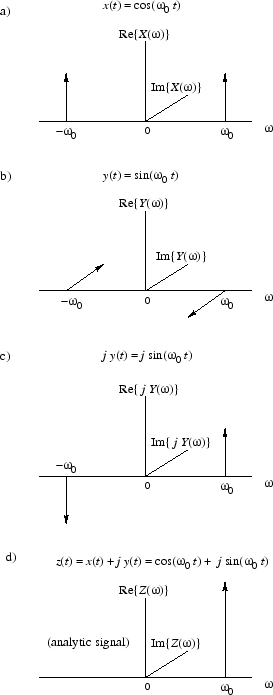Figure 4.16 illustrates what is going on in the frequency domain. At the top is a graph of the spectrum of the sinusoid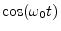consisting of impulses at frequencies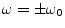and zero at all other frequencies (since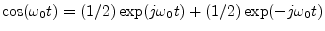). Each impulse amplitude is equal to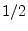. (The amplitude of an impulse is its algebraic area.) Similarly, since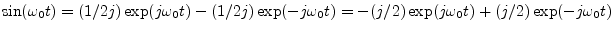, the spectrum of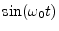is an impulse of amplitude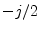at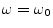and amplitude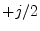at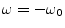. Multiplying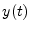by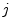results in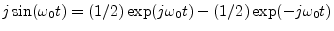which is shown in the third plot, Fig.4.16c. Finally, adding together the first and third plots, corresponding to, we see that the two positive-frequency impulses add in phase to give a unit impulse (corresponding to), and at frequency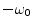, the two impulses, having opposite sign, cancel in the sum, thus creating an analytic signal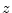, as shown in Fig.4.16d. This sequence of operations illustrates how the negative-frequency component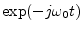gets filtered out by summingwith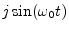to produce the analytic signalcorresponding to the real signal.

As a final example (and application), let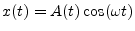, where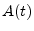is a slowly varying amplitude envelope (slow compared with). This is an example of amplitude modulation applied to a sinusoid at carrier frequency''(which is where you tune your AM radio). The Hilbert transform is very close to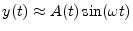(ifwere constant, this would be exact), and the analytic signal is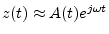. Note that AM demodulation4.14is now nothing more than the absolute value. I.e.,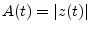. Due to this simplicity, Hilbert transforms are sometimes used in making amplitude envelope followers for narrowband signals (i.e., signals with all energy centered about a single carrier'' frequency). AM demodulation is one application of a narrowband envelope follower.

Next  |  Prev  |  Up  |  Top  |  Index  |  JOS Index  |  JOS Pubs  |  JOS Home  |  Search

[How to cite this work] [Order a printed hardcopy]